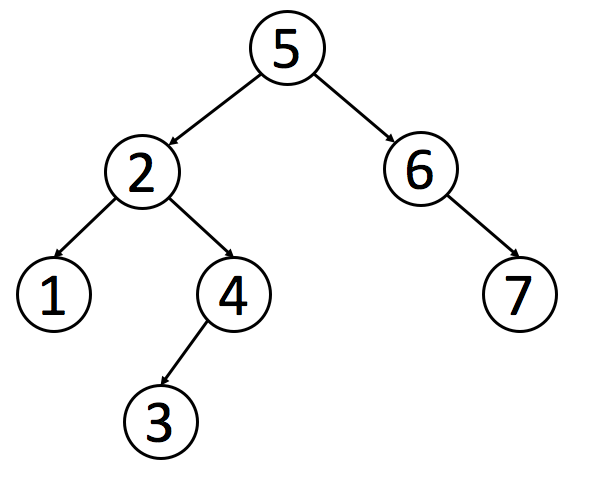## 前序遍历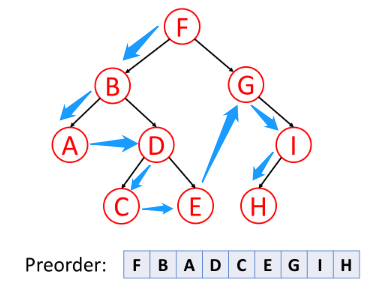``````// 定义二叉树节点
function TreeNode(val) {
this.val = val;
this.left = this.right = null;
}

// 递归
function preorderTraversal(root) {
if (!root) {
return []
}
let res = []
res.push(root.val)
root.left && res.push(...preorderTraversal(root.left))
root.right && res.push(...preorderTraversal(root.right))
return res
}

// 迭代，利用栈先进后出
function preorderTraversal(root) {
if (!root) {
return []
}
let stack = [], res = []
while(root) {
res.push(root.val)
// 后遍历右子树，先入栈
root.right && stack.push(root.right)
// 先遍历左子树，后入栈
root.left && stack.push(root.left)
// 左子树出栈
root = stack.pop()
}
return res
}
``````

## 中序遍历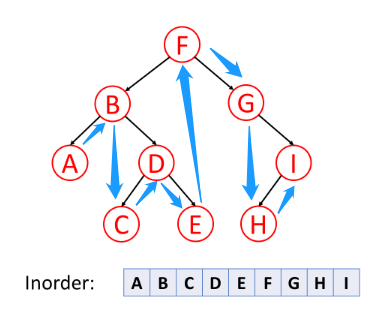1. 判断 left 节点是否存在，如果存在则进入 left 节点，并将当前点入栈
2. 当 left 节点和 right 节点都为 null 时，则记录当前节点，通过栈返回上一节点，将上一节点 left 置为 null
3. 当 left 节点为 null 且 right 节点存在时，则记录当前节点，然后跳到 right 节点
``````// 定义二叉树节点
function TreeNode(val) {
this.val = val;
this.left = this.right = null;
}

function inorderTraversal(root) {
let stack = [], res = []
while (root) {
if (root.left) {
stack.push(root)
root = root.left
} else if (!root.left && !root.right) {
res.push(root.val)
root = stack.pop()
root && (root.left = null)
} else if (root.right) {
res.push(root.val)
root = root.right
}
}
return res
}

function inorderTraversal(root) {
let stack = [], res = []
while (stack.length || root != null) {
while (root != null) {
stack.push(root)
root = root.left
}
root = stack.pop()
res.push(root.val)
root = root.right
}
return res
}
``````

## 后序遍历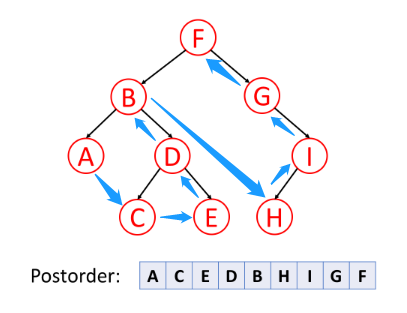1. 如果 left 节点存在，则遍历，直到末尾节点，才记录，然后返回上一节点
2. 当子节点遍历完毕时，才记录当前节点
``````// 定义二叉树节点
function TreeNode(val) {
this.val = val;
this.left = this.right = null;
}

function postorderTraversal(root) {
let stack = [], res = []
while(root) {
if (root.left) {
stack.push(root)
root = root.left
} else if (root.right) {
stack.push(root)
root = root.right
} else {
res.push(root.val)
root = stack.pop()
if (root && root.left) {
root.left = null
} else if (root && root.right) {
root.right = null
}
}
}
return res
}

// 类似前序遍历方法
function postorderTraversal(root) {
let stack = [], res = []
while(root) {
res.unshift(root.val)
root.left && stack.push(root.left)
root.right && stack.push(root.right)
root = stack.pop()
}
return res
}
``````

## 层序遍历``````// 定义二叉树节点
function TreeNode(val) {
this.val = val;
this.left = this.right = null;
}

function levelOrder(root) {
let queue = [], res = []
if (root) {
queue.push(root)
}
while (queue.length !== 0) {
let arr = [], len = queue.length
for (let i = 0; i < len; i++) {
let currNode = queue.shift()
if (currNode.left) {
queue.push(currNode.left)
}
if (currNode.right) {
queue.push(currNode.right)
}
arr.push(currNode.val)
}
res.push(arr)
}
return res
}
``````

## 运用递归解决树的问题

### “自顶向下” 的解决方案

“自顶向下” 意味着在每个递归层级，我们将首先访问节点来计算一些值，并在递归调用函数时将这些值传递到子节点。 所以 “自顶向下” 的解决方案可以被认为是一种前序遍历。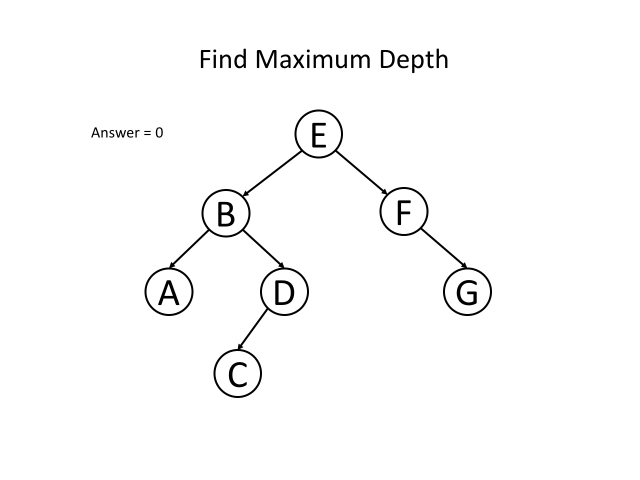### “自底向上” 的解决方案

“自底向上” 是另一种递归方法。 在每个递归层次上，我们首先对所有子节点递归地调用函数，然后根据返回值和根节点本身的值得到答案。 这个过程可以看作是后序遍历的一种。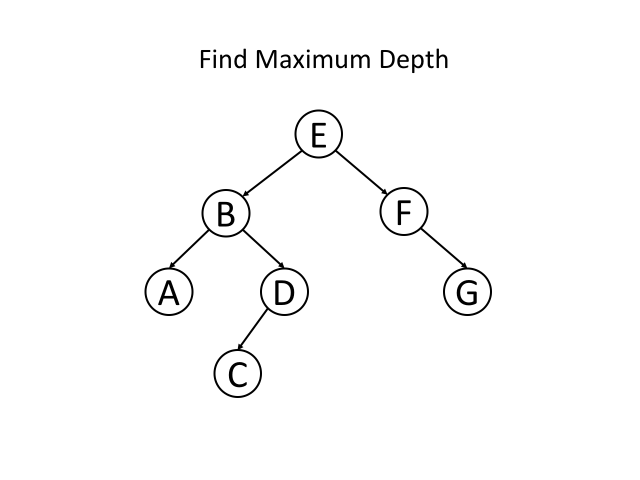### 总结

1. 你能确定一些参数，从该节点自身解决出发寻找答案吗？
2. 你可以使用这些参数和节点本身的值来决定什么应该是传递给它子节点的参数吗？ 如果答案都是肯定的，那么请尝试使用 “自顶向下” 的递归来解决此问题。

## 二叉树的最大深度

``````/**
* Definition for a binary tree node.
* function TreeNode(val) {
*     this.val = val;
*     this.left = this.right = null;
* }
*/
/**
* @param {TreeNode} root
* @return {number}
*/
var maxDepth = function(root) {
let depth = 0, queue = []
if (root) {
queue.push(root)
}
while(queue.length !== 0) {
depth += 1
let len = queue.length
for (let i = 0; i < len; i++) {
let currNode = queue.shift()
if (currNode.left) {
queue.push(currNode.left)
}
if (currNode.right) {
queue.push(currNode.right)
}
}
}
return depth
};
``````

## 重建二叉树

``````/* function TreeNode(x) {
this.val = x;
this.left = null;
this.right = null;
} */
function reConstructBinaryTree(pre, vin)
{
if (pre.length === 0 || vin.length === 0) {
return []
}
let node = new TreeNode(pre)
let i = 0
for (i; i < pre.length; i++) {
if (pre[i] == node.val) {
break
}
}
node.left = reConstructBinaryTree(pre.slice(1, i+1), vin.slice(0, i))
node.right = reConstructBinaryTree(pre.slice(1+1), vin.slice(i+1))
return node
}
``````

## 深度优先遍历 (DFS)

Depth-First-Search

## 广度优先遍历 (BFS)